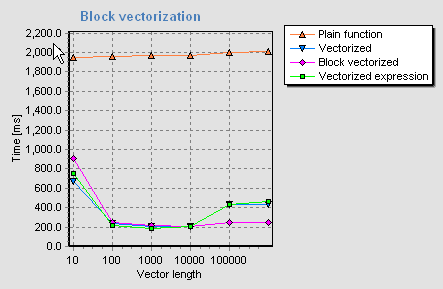# Block processing

Second step of code optimization after applying code vectorization is called block processing

When working with vectors it is absolutely critical to also consider the size of the CPU cache. If the arrays will not fit in the available CPU cache, a large (sometimes up to 3x) performance penalty will be imposed upon the algorithm. This means that vector arithmetic's should not be applied to vectors whose size exceed certain maximum length. Typically the maximum number of double precision elements ranges from 800 to 2000 per array. Longer vectors have to be split in pieces and processed in parts. MtxVec provides tools that allow you to achieve that easily. The following listing shows three versions of the same function.

Plain function:

`function MaxwellPDF(x, a: TSample): TSample;var xx: TSample;begin if (x >= 0) and (a > 0) then begin xx := Sqr(x); Result := Sqrt(4*a*INVTWOPI)*a*xx*Exp(-0.5*a*xx); end else Result := NAN;end;`

Vectorized function:

`procedure MaxwellPDF(X: TVec; a: TSample; Res: TVec);var Res1: TVec;begin CreateIt(Res1); try Res1.Sqr(X); Res.Copy(Res1); Res.Scale(-0.5*a); Res.Exp; Res.Mul(Res1); Res.Scale(Sqrt(4*a*INVTWOPI)*a); finally FreeIt(Res1);end;end;`On P4 2.4 GHz CPU the vectorized function is about 9.5x faster than the plain function when using Delphi 6. The block vectorized version of the function is a little slower for short vectors but maintains its high performance even for vectors exceeding 10 000 double precision elements. (For a CPU with 512kB CPU cache the limit is about 10 000 elements and if CPU with 128kB cache is used the limit is about 2000 elements.)

The block vectorized function is only marginally faster than vectorized version due to the use of SSE2 instructions. If the CPU does not support SSE, then the gain of the block vectorized version will be much more significant (typical gains are about 6 times). For example, when using older CPU's the speed of the plain function for vectors with length larger than the size of the CPU cache will be higher than that of its vectorized version. The vectorized version has to access memory multiple times, while the plain function version can cache some intermediate results in to FPU registers or CPU cache. The block vectorized version will ensure that the chunk of the vector being processed can fit in to the CPU cache and will thus give optimal performance for long vectors even in that case.